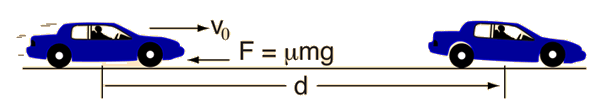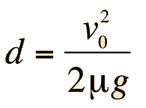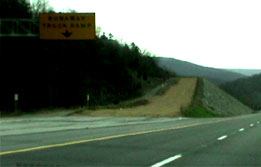# Stopping Distance for Auto

Assuming proper operation of the brakes, the minimum stopping distance for an automobile is determined by the effective coefficient of friction between the tires and the road. The friction force of the road must do enough work on the car to reduce its kinetic energy to zero (work-energy principle). If the wheels of the car continue to turn while braking, then static friction is operating, while if the wheels are locked and sliding over the road surface, the braking force is a kinetic friction force.To reduce the kinetic energy to zero:so the stopping distance isShow calculation
Note that this implies a stopping distance independent of vehicle mass. It also implies a quadrupling of stopping distance with a doubling of vehicle speed.Even though the stopping distance for a large truck should be the same as that for a car, there are real-world cases where a truck has more difficulty stopping. Runaway truck ramps are placed on long downgrades like this one on Monteagle in Tennessee. Deep, loose gravel provides a stopping force greater than that obtained from just a tire against the road.
Index

Work-energy principle

 HyperPhysics***** Mechanics R Nave
Go Back

# Stopping Distance Calculation

For calculating minimum stopping distance, a value of 0.8 is a nominal value for the coefficient of static friction between good tires and a good road surface. Generally, coefficients of kinetic friction are less, and may be dramatically less for wet, icy, or oily surfaces. For many existing tires, the coefficient of kinetic friction on a dry road surface may approach 0.8 if the braking is not so prolonged as to cause tire melting.The stopping distance is given by:Develop expression
If the vehicle speed is v = m/s = ft/s = km/hr = mi/hr
 and the coefficient of friction between the tires and the road is μ = ,
the stopping distance is d = m = ft.
You may enter data in any of the boxes above. The quantity will be calculated in the other unit systems. Then click on the active text for either stopping distance or vehicle speed to calculate that quantity. The quantities will not be forced to be consistent until you click on the quantity to be calculated.
Note that this calculation implies a stopping distance independent of vehicle mass. It also implies a quadrupling of stopping distance with a doubling of vehicle speed.

One experiment with trained drivers asked the drivers to stop a vehicle on signal by 1) locking the wheels and 2) stopping as fast as possible without locking the wheels. On dry flat concrete, the stopping distances were very nearly the same. Both test yielded coefficients of friction near 0.8 for tires with new tread on this surface. The coefficient of kinetic friction is anticipated to be considerably less on a wet surface where the water can act as a lubricant. It is also likely that worn tires will have a considerably smaller coefficient of kinetic friction than static friction.

Index

Work-energy principle

 HyperPhysics***** Mechanics R Nave
Go Back